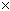From: Fadwa what is the greatest common factor(GCF) of the following algebraic expressions? 1680 and 6048 Hi Fadwa. You can always find the GCF if you break the two numbers down to their prime factors. A fundamental theorem holds that each number has only one prime factorization. For example, the prime factorization of 1680 is 24357 and the prime factorization of 6048 is 25337 . The GCF of a pair of numbers is always the product of the prime factors they have in common. Hope this helps, Stephen La Rocque.